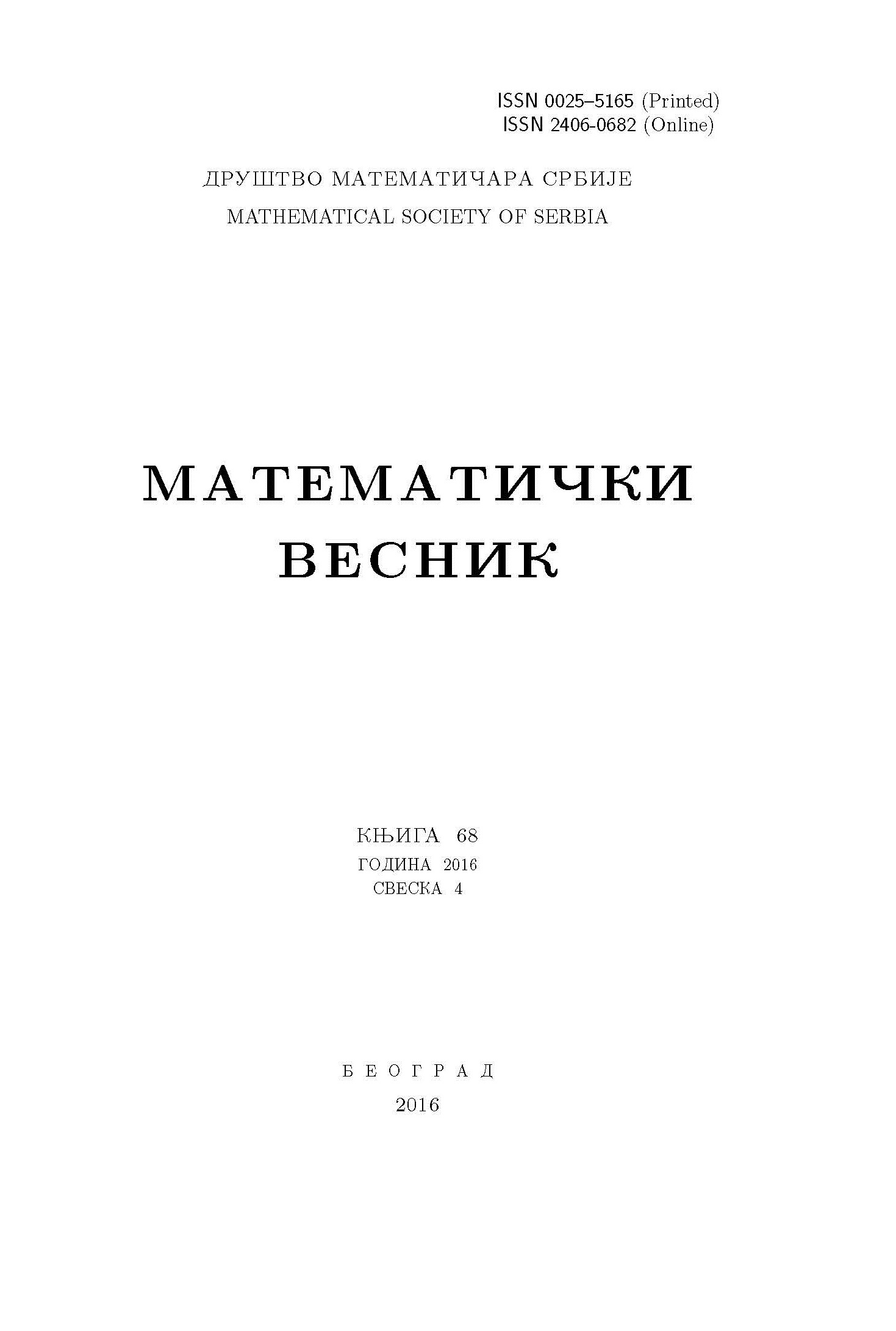﻿ Matematički Vesnik ﻿
 MATEMATIČKI VESNIK МАТЕМАТИЧКИ ВЕСНИКMODIFIED INERTIAL HYBRID SUBGRADIENT EXTRAGRADIENT METHOD FOR SOLVING VARIATIONAL INEQUALITIES AND FIXED POINT PROBLEMS FOR AN INFINITE FAMILY OF MULTIVALUED RELATIVELY NONEXPANSIVE MAPPINGS IN BANACH SPACES WITH APPLICATIONS T. O. Alakoya, O. T. Mewomo AbstractOne of the most interesting and important problems in the theory of variational inequalities is the study of efficient iterative schemes for finding approximate solutions and the convergence analysis of algorithms. In this article, we introduce a new inertial hybrid subgradient extragradient method for approximating a common solution of monotone variational inequalities and fixed point problems for an infinite family of relatively nonexpansive multivalued mappings in Banach spaces. In our proposed method, the projection onto the feasible set is replaced with a projection onto certain half spaces, which makes the algorithm easy to implement. We incorporate inertial term into the algorithm, which helps to improve the rate of convergence of the proposed method. Moreover, we prove a strong convergence theorem and we apply our results to approximate common solutions of variational inequalities and zero point problems, and to finding a common solution of constrained convex minimization and fixed point problems in Banach spaces. Finally, we present a numerical example to demonstrate the efficiency and the advantages of the proposed method, and we compare it with some related methods. Our results extend and improve some recent works both in Hilbert spaces and Banach spaces in this direction.Keywords: Inertial algorithm; hybrid subragradient extragradient method; variational inequality; fixed point problem; multivalued relatively nonexpansive mappings; zero point problems; convex minimization problems. MSC: 65K15, 47J25, 65J15, 90C33 Pages:  19$-$36 Volume  75 ,  Issue  1 ,  2023

﻿